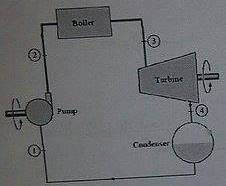# Thermodynamics: Steam Plant

## Homework Statement

The steam enter the turbine (3) of the steam plant at 8 MPa and 500°C. The steam extends in an isentropic process and leaves the turbine (4) at 200 kPa, then enters and leaves the condenser at constant pressure (You may treat the steam as a saturated liquid when it leaves the condenser). If the work of the pump is 7 kj/kg and both the kinetic and the potential energies are negligible. Compute:
1- The states of the steam when it enters and leaves the turbine.
2- The work done by the turbine in kj/kg.
3- The net power of the plant if the steam flows with 30 kg/s.
4- The amount of heat transfer of both the boiler and the condenser.
5- The efficiency of the cycle.
6- The two processes (3-4, 4-1) plotted on T-s diagram.## Homework Equations

Q-W=m(Δh)
efficiency=Wnet/Qin * 100
∑Qnet = ∑ Wnet

## The Attempt at a Solution

1-[/B] P3=8 MPa, T3=500°C
Using Tables: T3>TS ----> The inlet state is superheated vapor.
P4=200 kPa, s3=s4=6.7240 kg/kgK (Isentropic Process)
Using Tables: sf < s4 < sg ---> The exit state is mixture of saturated liquid and vapor.

2- Since q3-4=0 in the turbine, and both KE and PE are negligible, we get:
W3-4=h3-h4 (The first law of thermodynamics, h is the enthalpy)
h3= 3398.3 kj/kg, h4=2545.86 kj/kg
W3-4=652.44 kj/kg

3- Wnet=W3-4+Wpump=652.44+7=659.44 kj/kg
Wnet=Wnet * 30 kg/s = 19783.2 kj/s

4- Since W4-1=0 in the condenser, then q4-1=h1-h4
h1=504.7 kj/kg, h4=2545.86 kj/kg
q4-1=-2041.6 kj/kg.

Since qnet=Wnet --> The law of closed cycle
q4-1+qboiler=Wnet
qboiler=2701.04 kj/kg

5- eff. = Wnet/qboiler * 100 = 24.41%

6- http://i.imgur.com/xXeDpu8.jpg

Last edited:

I'm not sure whether I'm applying the first law for closed cycle right or not (∑Qnet = ∑ Wnet). Should I take the signs in the account or not?

SteamKing
Staff Emeritus
Homework Helper
Check the arithmetic on your calculation of W3-4.

Your overall thermal efficiency looked on the low side, and this may be part of the problem.

I can't what's wrong with W3-4. You know the first law of thermodynamics (for open systems) states:
Q-W=m(Δh+ΔKE+ΔPE) [kj/s]
Dividing both sides by m
q-w=(Δh+ΔKE+ΔPE) [kj/kg]
Since q=ΔKE=ΔPE=0
-w=Δh --> w=-Δh --> w=h1-h2
Isn't that right?

SteamKing
Staff Emeritus
Homework Helper
I can't what's wrong with W3-4. You know the first law of thermodynamics (for open systems) states:
Q-W=m(Δh+ΔKE+ΔPE) [kj/s]
Dividing both sides by m
q-w=(Δh+ΔKE+ΔPE) [kj/kg]
Since q=ΔKE=ΔPE=0
-w=Δh --> w=-Δh --> w=h1-h2
Isn't that right?
It isn't the thermodynamics that's the problem, it's the arithmetic (you know, addition, subtraction, etc.) Check you calculation...
h3= 3398.3 kj/kg, h4=2545.86 kj/kg
W3-4=652.44 kj/kg

Is 3398.3 - 2545.86 = 652.44? That's what needs checking.

Okay, my bad ^^", but the approach is correct, right?

SteamKing
Staff Emeritus
Homework Helper
Okay, my bad ^^", but the approach is correct, right?
Yes, but you still must be careful of errors in calculation. Correct your mistake and re-post your work.

W3-4=852.44 kJ/kg
Wnet=859.44 kj/kg
Wnet=2.5783e04 kj/s
qboiler=2.901e03
eff. = Wnet/qboiler * 100 = 29.63%

SteamKing
Staff Emeritus
Homework Helper
W3-4=852.44 kJ/kg
Wnet=859.44 kj/kg
W`net=2.5783e04 kj/s
qboiler=2.901e03
eff. = Wnet/qboiler * 100 = 29.63%
The net work is what comes out of the turbine, W3-4, not the turbine + feed pump. You must supply the energy to run the feed pump from some external source, thus this is work which must be input to the system, and not work which is coming out of the system.

The thermal efficiency of the cycle is based on this value of net work, W3-4.

The energy which is used to raise the pressure of the feed water to return it to the boiler is added to the enthalpy of the condensate coming out of the condenser, and thus reduces slightly the amount of heat input which must be furnished by the boiler.

SteamKing
Staff Emeritus